Cones and their plane development

A cone is a geometric body consisting of a plane base bounded by a closed curve (the directrix) and every point of this curve is joined to a fixed point (the apex or vertex) lying outside the plane of the base. A pyramid is a special case of a cone with a polygonal base. If the directrix is a circle and the apex is perpendicularly above the center of the circle then the cone is a right circular cone. Then the cone has a rotational symmetry around the straight line passing through the apex (the axis of the cone). Each of the line segments between the apex and the base circle is a generatrix.

The main interest of this page is to see how right circular cones can be developed into a plane.

This is a right circular cone:The cone developing into a plane: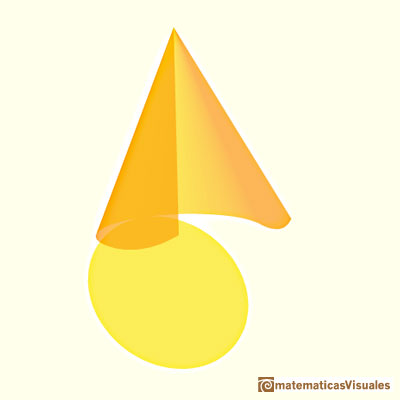This is a plane development of a cone: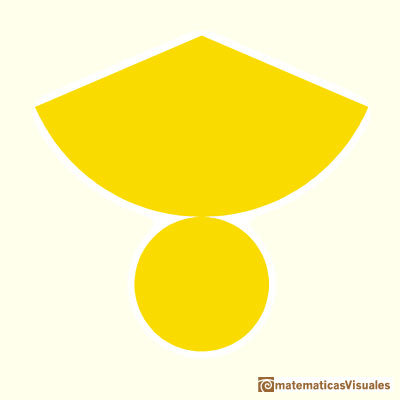To calculate the lateral surface area of a cone we need the slant height. The slant height is the distance from the base circle to the apex of the cone (the generatrix as a segment). There is a relation between the slant height and the height of a cone (Pythagorean theorem).We are going to calculate the lateral surface area of a cone that is the area of a circular sector. If R is the base radius, the formula for the lateral surface area of a cone is like the formula for the area of a triangle. (The intuitive reason is like Kepler in Kepler and the area of a circle):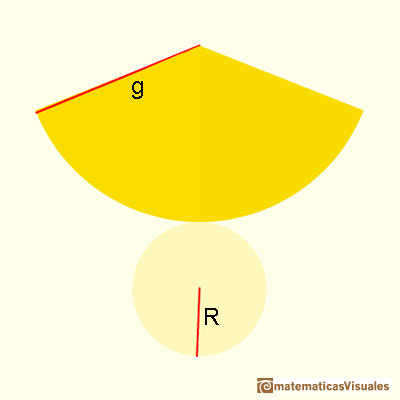Do you remember the formula for the volume of a cone?

A cone with its apex cut off by a plane is called a truncated cone. If this truncation plane is parallel to the base then the body is called a conical frustum.

For example, this is a conical frustum:A conical frustum developing into a plane:And this is its plane development: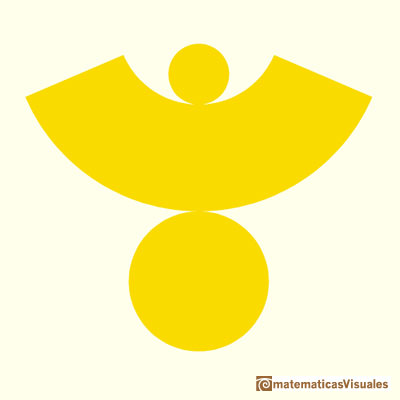As before, we need the slant height to calculate the lateral surface area of a frustum:We can think, intuitively, that a cylindrical frustum is like a pyramidal frustum "with an infinite number of lateral faces". This is a very imprecise way of thinking that can remind us the origins of the Calculus, like Kepler's era. We can remind that the formula for the lateral surface area of a pyramidal frustum is like the area of a trapezoid (lateral faces are congruent trapezoids). When we calculate the lateral surface area of a conical frustum, the formula reminds us the formula for the trapezoid again: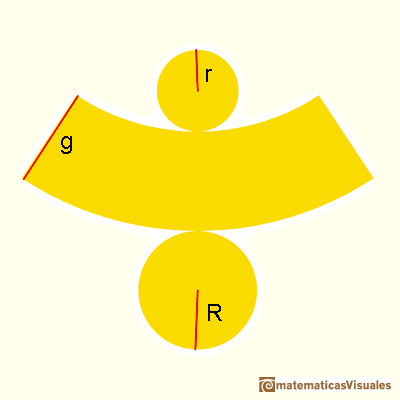Door in Peñalba de Santiago (León, Spain, July 2016)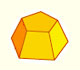Plane net of pyramids and pyramidal frustrum. How to calculate the lateral surface area.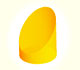We study different cylinders cut by an oblique plane. The section that we get is an ellipse.We study different cylinders and we can see how they develop into a plane. Then we explain how to calculate the lateral surface area.The section of a cylinder by a plane cutting its axis at a single point is an ellipse. A beautiful demonstration uses Dandelin Spheres.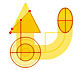Durer was the first who published in german a method to draw ellipses as cone sections.Durer made a mistake when he explanined how to draw ellipses. We can prove, using only basic properties, that the ellipse has not an egg shape .Plane nets of prisms with a regular base with different side number cut by an oblique plane.We study different prisms and we can see how they develop into a plane net. Then we explain how to calculate the lateral surface area.The first drawing of a plane net of a regular dodecahedron was published by Dürer in his book 'Underweysung der Messung' ('Four Books of Measurement'), published in 1525 .The first drawing of a plane net of a regular octahedron was published by Dürer in his book 'Underweysung der Messung' ('Four Books of Measurement'), published in 1525 .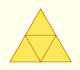The first drawing of a plane net of a regular tetrahedron was published by Dürer in his book 'Underweysung der Messung' ('Four Books of Measurement'), published in 1525 .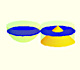Using Cavalieri's Principle we can calculate the volume of a sphere.The volume of a tetrahedron is one third of the prism that contains it.The first drawing of a plane net of a regular tetrahedron was published by Dürer in his book 'Underweysung der Messung' ('Four Books of Measurement'), published in 1525 .The volume of an octahedron is four times the volume of a tetrahedron. It is easy to calculate and then we can get the volume of a tetrahedron.You can chamfer a cube and then you get a polyhedron similar (but not equal) to a truncated octahedron. You can get also a rhombic dodecahedron.